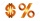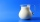# Fractions - 8th grade (13y) - math problems

#### Number of problems found: 260

• Equation with fractionsSolve equation: ? It is equation with fractions.
• Equation with mixed fractions2 3/5 of 1430+? = 1900. How to do this problem
• FractionsThree-quarters of an unknown number are 4/5. What is 5/6 of this unknown number?
• InqualitySolve inequality: 3x + 6 > 14
• What percentWhat percent of 51 3/5 is 47 2/5?
• Value 4If 5/18=425, what is the value 13/18?
• Simple equation 6Solve equation with one variable: X/2+X/3+X/4=X+4
• Equation with xSolve the following equation: 2x- (8x + 1) - (x + 2) / 5 = 9
• Find the 11Find the quotient of 229.12 and 12.32
• Percentage - fractionsAbout what percentage we must increase number 1/6 to get number 1/3?
• A bucketA bucket has 4 liters of water in it when it is 2/5 full. How much can it hold?
• 3/8 offA shirt cost 38.95 How much will you pay for the shirt if it has a discount tag for 3/8 off? Round to the nearest cent
• Percentages 5\$5.25 is 7 1/2% of what number?
• In centerWhat number lies in the center between a quarter of a fifth and a half of a third on the number axis?
• Mixing 5Carlos mixed 4/15 of chocolate syrup with 1/2 of milk. Determine the reasonable estimate of the total amount of liquid
• Two numbersThe sum of the two numbers is 1. Find both numbers if you know that half of the first is equal to one seventh of the second number.
• UN 1If we add to an unknown number his quarter, we get 210. Identify unknown number.
• Class 8.AThree quarters of class 8.A went skiing. Of those who remained at home one third was ill and the remaining six were on math olympic. How many students have class 8.A?
• Marriage sttusIn our city, there are 3/5 of the women married to 2/3 of the men. Find what part of the population is free.
• An airplaneAn airplane flies 1440 km in 2 1/4 hours. What is its average speed in km per hour?

Do you have an interesting mathematical word problem that you can't solve it? Submit a math problem, and we can try to solve it.

We will send a solution to your e-mail address. Solved examples are also published here. Please enter the e-mail correctly and check whether you don't have a full mailbox.

Please do not submit problems from current active competitions such as Mathematical Olympiad, correspondence seminars etc...

Need help calculate sum, simplify or multiply fractions? Try our fraction calculator.